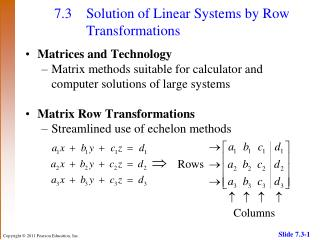# 7.3 Solution of Linear Systems by Row Transformations - PowerPoint PPT PresentationDownload Presentation7.3 Solution of Linear Systems by Row Transformations

7.3 Solution of Linear Systems by Row Transformations
Download Presentation## 7.3 Solution of Linear Systems by Row Transformations

- - - - - - - - - - - - - - - - - - - - - - - - - - - E N D - - - - - - - - - - - - - - - - - - - - - - - - - - -
##### Presentation Transcript

1. 7.3 Solution of Linear Systems by Row Transformations • Matrices and Technology • Matrix methods suitable for calculator and computer solutions of large systems • Matrix Row Transformations • Streamlined use of echelon methods

2. 7.3 Solution of Linear Systems by Row Transformations • This is called an augmented matrix where each member of the array is called an element or entry. • The rows of an augmented matrix can be treated just like the equations of a linear system.

3. 7.3 Matrix Transformations • For any augmented matrix of a system of linear • equations, the following row transformations will • result in the matrix of an equivalent system. • Any two rows may be interchanged. • The elements of any row may be multiplied by a nonzero real number. • Any row may be changed by adding to its elements a multiple of the corresponding elements of another row.

4. 7.3 Solving a System by the Row Echelon Method Example Solve the system. Analytic Solution The augmented matrix for the system is

5. 7.3 Solving a System by the Row Echelon Method The matrix represents the system The solution to this system using back-substitution is {(1, –2)}.

6. 7.3 Solving a System by the Row Echelon Method Graphing Calculator Solution Enter the augmented matrix of the system. Using the ref command on the TI-83, we can find the row echelon form of the matrix.

7. 7.3 Solving a System by the Row Echelon Method The left screen displays decimal entries, but can be converted to fractions as indicated in the right screen. This corresponds to the augmented matrix in the analytic solution. The rest of the solution process is the same.

8. 7.3 Extending the Row Echelon Method to Larger Systems Example Solve the system. The augmented matrix of the system is

9. 7.3 Extending the Row Echelon Method to Larger Systems There is already a 1 in row 1, column 1. Next, get 0s in the rest of column 1.

10. 7.3 Extending the Row Echelon Method to Larger Systems

11. 7.3 Extending the Row Echelon Method to Larger Systems The corresponding matrix to the system is Applying back-substitution we get the solution {(1, 2, –1)}.

12. 7.3 Reduced Row Echelon Method • Matrix methods for solving systems • Row Echelon Method form of a matrix seen earlier • 1s along the diagonal and 0s below • Reduced Row Echelon Method form of a matrix • 1s along the diagonal and 0s both below and above

13. 7.3 Reduced Row Echelon Method Example The augmented form of the system is Using the row transformations, this augmented matrix can be transformed to

14. 7.3 Reduced Row Echelon Method with the Graphing Calculator Example Solve the system. Solution The augmented matrix of the system is shown below.

15. 7.3 Reduced Row Echelon Method with the Graphing Calculator Using the rref command we obtain the row reduced echelon form.

16. 7.3 Solving a System with No Solutions Example Show that the following system is inconsistent. Solution The augmented matrix of the system is

17. 7.3 Solving a System with No Solutions The final row indicates that the system is inconsistent and has solution set .

18. 7.3 Solving a System with Dependent Equations Example Show that the system has dependent equations. Express the general solution using an arbitrary variable. Solution The augmented matrix is

19. 7.3 Solving a System with Dependent Equations The final row of 0s indicates that the system has dependent equations. The first two rows represent the system

20. 7.3 Solving a System with Dependent Equations Start with the augmented matrix

21. 7.3 Solving a System with Dependent Equations The equations that correspond to the final matrix are

22. 7.3 Solving a System with Dependent Equations Solving for y we get Substitute this result into the expression to find x. Solution set written with z arbitrary: